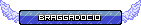# Kramuals explained##Kramuals explained

So over at the livestream we had a discussion on why kramuals are possible, and why diagonal kramuals aren't, and to so explain this, here I am with the explanation.

So first up, the snippet of code that makes it work:

Code:
`function satisfyDistance()    {        var _loc3 = a.x - b.x;        var _loc2 = a.y - b.y;        var _loc7 = Math.sqrt(_loc3 * _loc3 + _loc2 * _loc2);        var _loc6 = (_loc7 - restLength) / _loc7 * 5.000000E-001;        var _loc5 = _loc3 * _loc6;        var _loc4 = _loc2 * _loc6;        a.x = a.x - _loc5;        a.y = a.y - _loc4;        b.x = b.x + _loc5;        b.y = b.y + _loc4;    } // End of the function`

This code here (and variation of others) exist in bosh's "skeleton" physics. These "skeleton" connections are what makes bosh sit upright, and return back to "normal" position after being left alone for a certain amount of time. For a visual representation, you can turn on contact points with my builds, or use 6.1 contact points to view every skeletal connection bosh has.

What this function does is basically check the distance between each contact point and tries to move him back to the default distance, not position, distance.

What each thing means:
a.x/a.y = is one contact point's coordinates, b.x/b.y = second contact point's coordinates. Everything else isn't as significant.

Now here's how a kramual occurs. Look at these two lines:

Code:
`var _loc3 = a.x - b.x;var _loc2 = a.y - b.y;`

This is the initial step for checking the distance between the two points. If the math behing var _loc3 or var _loc2 result in zero, a kramual may occur. Now, assuming that only one of these results in 0, I shal shift your attention to the second part of this code:

Code:
`var _loc5 = _loc3 * _loc6;var _loc4 = _loc2 * _loc6;`

Notice how it's asking for _loc3 or _loc2 to be MULTIPLIED by whatever _loc6 is? Don't worry about whatever _loc6 is, we all know anything multiplied by zero, equals 0. So if _loc3 is 0, _loc5 is 0. And if _loc2 is 0, _loc4 is 0. No questions asked.

And finally, the third snippet of code:

Code:
`a.x = a.x - _loc5;a.y = a.y - _loc4;b.x = b.x + _loc5;b.y = b.y + _loc4;`

Notice how it's trying to add _loc4 and _loc5 to the XY positions of the points? Well, what happens if you add 0 to the coordinate? It doesn't move. And if every point ends up sharing the X or Y coordinate, kramual happens. This doesn't mean that bosh stops moving, we all know you can keep bosh moving in any direction in a kramual. What it means is that the points won't stop sharing the same coordinates, IE that kramual lock we get. Hence why kramuals tend to crash only if rotated, or can only be broken from with a specific well, most commonly the shoulder, because you're forcing a change in the points position.

So why aren't diagonal kramuals possible? Simple. The X or Y points have to IDENTICAL, diagonal lines by default do not share X or Y positions anywhere on the line. The resulting math doesn't cause _loc2 or _loc3 to result in 0, and therefore the skeleton will be able to adjust the points as needed.

_________________
LR Subreddit http://www.reddit.com/r/linerider/
Discord Group https://discord.gg/0ggPNq98iruWoixw
OpenLR https://github.com/kevansevans/openLR

##Re: Kramuals explained

Not sure I toooootally understood that but I think I see why you say that diagonal kramuals are impossible now.

_________________
Support My Art
A playlist of my best tracks
To keep up with what I'm up to in the world of Line Rider, follow me:
My Twitter | My Tumblr | My Twitch | My Facebook Page | My Instagram
(You can also follow my patreon and see all public posts completely for free)rabid squirrel
Member

I'm the artsy person round here##Re: Kramuals explained

Fauxfyre
Member##Re: Kramuals explained